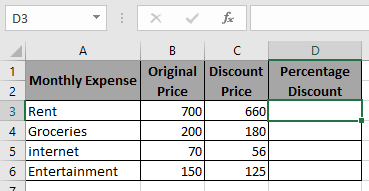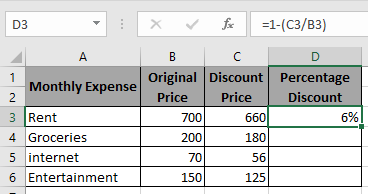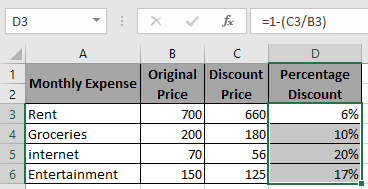# How to Calculate Percentage Discount in Excel

In this article, we will learn about how to calculate the percentage discount off the set of data provided in Excel.

Percentage discount calculates the change in Sale price and discount price.

Percentage Discount = 1 - (discount amount / original price)

We will use this in Excel to find Percentage change.

Here we will use the above percentage discount formula in the below example.Use the Formula in D3 cell

=1-(C3/B3)As you can see in the above snapshot first data percentage discount is 6%.

Copy the formula in the remaining cells to get the percentage discount for the rest of the data.Percentage discount can be calculated using the above method.

Hope you understood how to calculate the Percentage Discount of a set of values. Explore more articles on Mathematical formulation in Excel here. Mention your queries in the comment box below. We will help you with it.

Related Article:

How to Calculate Profit margin percentage in Excel

How to Percentage Decrease Change in Excel

How to Calculate Percentage of Total in Excel

How to Increase by percentage in Excel

Popular Articles:

50 Excel Shortcuts to Increase Your Productivity

How to use the VLOOKUP Function in Excel

How to use the COUNTIF function in Excel

How to use the SUMIF Function in Excel

Terms and Conditions of use

The applications/code on this site are distributed as is and without warranties or liability. In no event shall the owner of the copyrights, or the authors of the applications/code be liable for any loss of profit, any problems or any damage resulting from the use or evaluation of the applications/code.